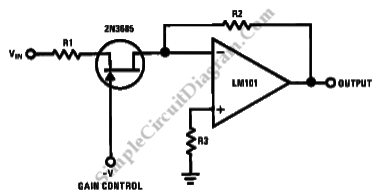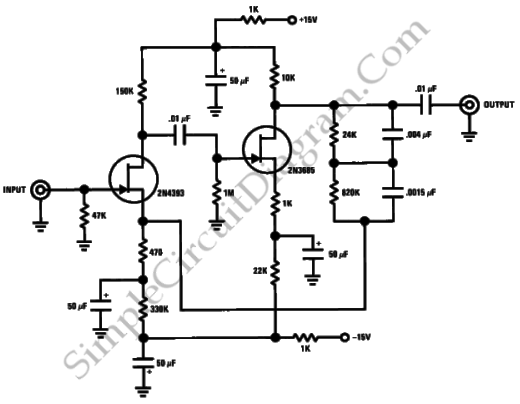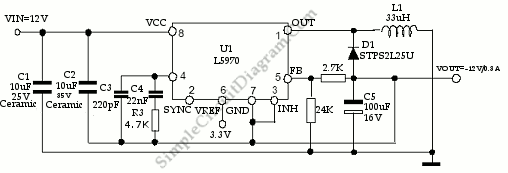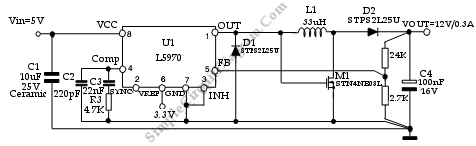## Variable AttenuatorThis is a variable attenuator circuit. This circuit can be used to reduce the power or amplitude of a signal without appreciably distorting its waveform. Here is the circuit : This circuit uses 2N3685 as a voltage variable resistor with an RDS(on) of 800 Ohm. Using 2N3685 JFET, this circuit will provide excellent electronic gain control because 2N3685 has linear […]

## Magnetic-Pickup Phono PreamplifierThis is a simple circuit of magnetic pickup phono preamplifier. A proper loading to a reluctance is provided by this circuit. Here is the circuit: The gain provided by this circuit is approximately 25dB at 1kHz (2.2mV input for 100mV output). Referenced to 10mV input at 1 kHz, the S +N/N ratio is better than -70dB and the the dynamic […]

## Switch Mode Regulator Provides -12V Negative Supply from Positive SupplyThis is a circuit that can provide -12 V negative supply from positive supply. The output voltage is Vo= -Vin· D/(1-D). The maximum output current is Iout=1·(1-D). Here is the circuit : In this circuit, the ground of U1 is connected to negative output voltage. The input voltage of U1 must be lower than maximum operating input voltage, 36V, because […]